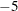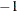The COPULA Procedure

BOUNDS Statement

• BOUNDS bound1 <, bound2 …>;

The BOUNDS statement specifies the lower and upper bounds for the parameters. You can use this statement only when maximum likelihood estimation is used for the specified copula. Each bound is composed of parameters, constants, and inequality operators in the following format:

operator item < operator item < operator item …>>

Each item is a constant, parameter, or list of parameters. Parameters associated with a regressor variable are referred to by the name of the corresponding regressor variable. Each operator is <, >, <=, or >=. The following example indicates that the lower and upper bounds for the parameter THETA areand 10, respectively.

```   bounds -5 < THETA < 10;
```

If you do not specify bounds, the internal default values are used; the default values are described in the section Details: COPULA Procedure. For the normal and t copulas, the correlation matrix uses only the default parameter bounds, which areand 1 for lower bound and upper bound, respectively.